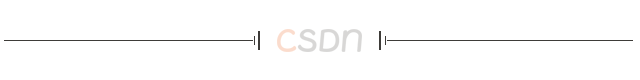【编者按】这是一篇关于机器学习工具包Scikit-learn的入门级读物。对于程序员来说，机器学习的重要性毋庸赘言。也许你还没有开始，也许曾经失败过，都没有关系，你将在这里找到或者重拾自信。只要粗通Python，略知NumPy，认真读完这21句话，逐行敲完示例代码，就可以由此进入自由的王国。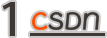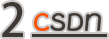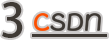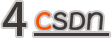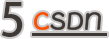``````>>> import numpy as np
>>> members = np.array([
['男', '25', 185, 80, '程序员', 35, 200,  30],
['女', '23', 170, 55, '公务员', 15,   0,  80],
['男', '30', 180, 82, '律师',   60, 260, 300],
['女', '27', 168, 52, '记者',   20, 180, 150]
])
``````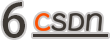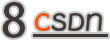``````>>> security = np.float32((members[:,-1])) # 提取有价证券特征列数据
>>> security
array([ 30.,  80., 300., 150.], dtype=float32)
>>> (security - security.mean())/security.std() # 减去均值再除以标准差
array([-1.081241, -0.5897678, 1.5727142, 0.09829464], dtype=float32)
``````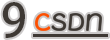###### 归一化是对样本集的每个特征列减去该特征列的最小值进行中心化，再除以极差（最大值最小值之差）进行缩放。

``````>>> security = np.float32((members[:,-1])) # 提取有价证券特征列数据
>>> security
array([ 30.,  80., 300., 150.], dtype=float32)
>>> (security - security.min())/(security.max() - security.min()) # 减去最小值再除以极差
array([0., 0.18518518, 1., 0.44444445], dtype=float32)
``````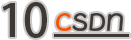``````>>> from sklearn import preprocessing as pp
>>> X = [
['男', '程序员'],
['女', '公务员'],
['男', '律师', ],
['女', '记者', ]
]
>>> ohe = pp.OneHotEncoder().fit(X)
>>> ohe.transform(X).toarray()
array([[0., 1., 0., 0., 1., 0.],
[1., 0., 1., 0., 0., 0.],
[0., 1., 0., 1., 0., 0.],
[1., 0., 0., 0., 0., 1.]])
``````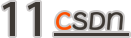• datasets.fetch_20newsgroups([data_home, …]) ：加载新闻文本分类数据集

• datasets.fetch_20newsgroups_vectorized([…]) ：加载新闻文本向量化数据集

• datasets.fetch_california_housing([…]) ：加载加利福尼亚住房数据集

• datasets.fetch_covtype([data_home, …]) ：加载森林植被数据集

• datasets.fetch_kddcup99([subset, data_home, …]) ：加载网络入侵检测数据集

• datasets.fetch_lfw_pairs([subset, …]) ：加载人脸（成对）数据集

• datasets.fetch_lfw_people([data_home, …]) ：加载人脸（带标签）数据集

• datasets.fetch_olivetti_faces([data_home, …]) ：加载 Olivetti 人脸数据集

• datasets.fetch_rcv1([data_home, subset, …])：加载路透社英文新闻文本分类数据集

• datasets.fetch_species_distributions([…]) ：加载物种分布数据集``````>>> from sklearn.datasets import load_iris
>>> X.shape # 数据集X有150个样本，4个特征列
(150, 4)
>>> y.shape # 标签集y的每一个标签和数据集X的每一个样本一一对应
(150,)
>>> X, y
(array([5.1, 3.5, 1.4, 0.2]), 0)
``````

``````>>> iris = load_iris()
>>> iris.target_names # 查看标签的名字
array(['setosa', 'versicolor', 'virginica'], dtype='<U10')
>>> X = iris.data
>>> y = iris.target
``````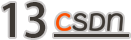``````>>> from sklearn.datasets import load_iris
>>> from sklearn.model_selection import train_test_split as tsplit
>>> X_train, X_test, y_train, y_test = tsplit(X, y, test_size=0.1)
>>> X_train.shape, X_test.shape
((135, 4), (15, 4))
>>> y_train.shape, y_test.shape
((135,), (15,))
``````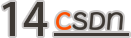k-近邻分类是最简单、最容易的分类方法。对于待分类的样本，从训练集中找出k个和它距离最近的样本，考察这些样本中哪一个标签最多，就给待分类样本贴上该标签。k值的最佳选择高度依赖数据，较大的k值会抑制噪声的影响，但同时也会使分类界限不明显。通常k值选择不大于20的整数。

``````>>> from sklearn.datasets import load_iris
>>> from sklearn.model_selection import train_test_split as tsplit
>>> from sklearn.neighbors import KNeighborsClassifier # 导入k-近邻分类模型
>>> X, y = load_iris(return_X_y=True) # 获取鸢尾花数据集，返回样本集和标签集
>>> X_train, X_test, y_train, y_test = tsplit(X, y, test_size=0.1) # 拆分为训练集和测试集
>>> m = KNeighborsClassifier(n_neighbors=10) # 模型实例化，n_neighbors参数指定k值，默认k=5
>>> m.fit(X_train, y_train) # 模型训练
KNeighborsClassifier()
>>> m.predict(X_test) # 对测试集分类
array([2, 1, 2, 2, 1, 2, 1, 2, 2, 1, 0, 1, 0, 0, 2])
>>> y_test # 这是实际的分类情况，上面的预测只错了一个
array([2, 1, 2, 2, 2, 2, 1, 2, 2, 1, 0, 1, 0, 0, 2])
>>> m.score(X_test, y_test) # 模型测试精度（介于0~1）
0.9333333333333333
``````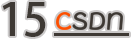k-近邻算法不仅可以用来解决分类问题，也可以用来解决回归问题。k-近邻回归预测样本的标签由它最近邻标签的均值计算而来。下面的代码以波士顿房价数据集为例，演示了k-近邻回归模型的用法。波士顿房价数据集统计的是20世纪70年代中期波士顿郊区房价的中位数，一共有506条不同的数据，每条数据包含区域的人文环境、自然环境、商业环境、交通状况等13个属性，标签是区域房价的平均值。

``````>>> from sklearn.datasets import load_boston
>>> from sklearn.model_selection import train_test_split as tsplit
>>> from sklearn.neighbors import KNeighborsRegressor
>>> X, y = load_boston(return_X_y=True) # 加载波士顿房价数据集
>>> X.shape, y.shape, y.dtype # 该数据集共有506个样本，13个特征列，标签集为浮点型，适用于回归模型
((506, 13), (506,), dtype('float64'))
>>> X_train, X_test, y_train, y_test = tsplit(X, y, test_size=0.01) # 拆分为训练集和测试集
>>> m = KNeighborsRegressor(n_neighbors=10) # 模型实例化，n_neighbors参数指定k值，默认k=5
>>> m.fit(X_train, y_train) # 模型训练
KNeighborsRegressor(n_neighbors=10)
>>> m.predict(X_test) # 预测6个测试样本的房价
array([27.15, 31.97, 12.68, 28.52, 20.59, 21.47])
>>> y_test # 这是测试样本的实际价格，除了第2个（索引为1）样本偏差较大，其他样本偏差还算差强人意
array([29.1, 50. , 12.7, 22.8, 20.4, 21.5])
``````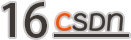``````>>> from sklearn import metrics
>>> y_pred = m.predict(X_test)
>>> metrics.mean_squared_error(y_test, y_pred) # 均方误差
60.27319999999995
>>> metrics.median_absolute_error(y_test, y_pred) # 中位数绝对误差
1.0700000000000003
>>> metrics.r2_score(y_test, y_pred) # 复相关系数
0.5612816401629652
``````

``````>>> from sklearn.datasets import load_boston
>>> from sklearn.model_selection import train_test_split as tsplit
>>> from sklearn.tree import DecisionTreeRegressor
>>> X, y = load_boston(return_X_y=True) # 加载波士顿房价数据集
>>> X_train, X_test, y_train, y_test = tsplit(X, y, test_size=0.01) # 拆分为训练集和测试集
>>> m = DecisionTreeRegressor(max_depth=10) # 实例化模型，决策树深度为10
>>> m.fit(X, y) # 训练
DecisionTreeRegressor(max_depth=10)
>>> y_pred = m.predict(X_test) # 预测
>>> y_test # 这是测试样本的实际价格，除了第2个（索引为1）样本偏差略大，其他样本偏差较小
array([20.4,  21.9,  13.8,  22.4, 13.1,  7. ])
>>> y_pred # 这是6个测试样本的预测房价，非常接近实际价格
array([20.14, 22.33, 14.34, 22.4, 14.62, 7. ])
>>> metrics.r2_score(y_test, y_pred) # 复相关系数
0.9848774474870712
>>> metrics.mean_squared_error(y_test, y_pred) # 均方误差
0.4744784865112032
>>> metrics.median_absolute_error(y_test, y_pred) # 中位数绝对误差
0.3462962962962983
``````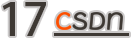``````>>> from sklearn.datasets import load_diabetes
>>> from sklearn.model_selection import train_test_split as tsplit
>>> from sklearn.svm import SVR
>>> from sklearn import metrics
>>> X.shape, y.shape, y.dtype
((442, 10), (442,), dtype('float64'))
>>> X_train, X_test, y_train, y_test = tsplit(X, y, test_size=0.02)
>>> svr_1 = SVR(kernel='rbf', C=0.1) # 实例化SVR模型，rbf核函数，C=0.1
>>> svr_2 = SVR(kernel='rbf', C=100) # 实例化SVR模型，rbf核函数，C=100
>>> svr_1.fit(X_train, y_train) # 模型训练
SVR(C=0.1)
>>> svr_2.fit(X_train, y_train) # 模型训练
SVR(C=100)
>>> z_1 = svr_1.predict(X_test) # 模型预测
>>> z_2 = svr_2.predict(X_test) # 模型预测
>>> y_test # 这是测试集的实际值
array([ 49., 317.,  84., 181., 281., 198.,  84.,  52., 129.])
>>> z_1 # 这是C=0.1的预测值，偏差很大
array([138.10720127, 142.1545034 , 141.25165838, 142.28652449,
143.19648143, 143.24670732, 137.57932272, 140.51891989,
143.24486911])
>>> z_2 # 这是C=100的预测值，偏差明显变小
array([ 54.38891948, 264.1433666 , 169.71195204, 177.28782561,
283.65199575, 196.53405477,  61.31486045, 199.30275061,
184.94923477])
>>> metrics.mean_squared_error(y_test, z_1) # C=0.01的均方误差
8464.946517460194
>>> metrics.mean_squared_error(y_test, z_2) # C=100的均方误差
3948.37754995066
>>> metrics.r2_score(y_test, z_1) # C=0.01的复相关系数
0.013199351909129464
>>> metrics.r2_score(y_test, z_2) # C=100的复相关系数
0.5397181166871942
>>> metrics.median_absolute_error(y_test, z_1) # C=0.01的中位数绝对误差
57.25165837797314
>>> metrics.median_absolute_error(y_test, z_2) # C=100的中位数绝对误差
22.68513954888364
``````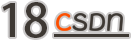``````>>> from sklearn.datasets import load_breast_cancer # 导入数据加载函数
>>> from sklearn.tree import DecisionTreeClassifier # 导入随机树
>>> from sklearn.ensemble import RandomForestClassifier # 导入随机森林
>>> from sklearn.model_selection import cross_val_score # 导入交叉验证
>>> ds = load_breast_cancer() # 加载威斯康星州乳腺癌数据集
>>> ds.data.shape # 569个乳腺癌样本，每个样本包含30个特征
(569, 30)
>>> dtc = DecisionTreeClassifier() # 实例化决策树分类模型
>>> rfc = RandomForestClassifier() # 实例化随机森林分类模型
>>> dtc_scroe = cross_val_score(dtc, ds.data, ds.target, cv=10) # 交叉验证
>>> dtc_scroe # 决策树分类模型交叉验证10次的结果
array([0.92982456, 0.85964912, 0.92982456, 0.89473684, 0.92982456,
0.89473684, 0.87719298, 0.94736842, 0.92982456, 0.92857143])
>>> dtc_scroe.mean() # 决策树分类模型交叉验证10次的平均精度
0.9121553884711779
>>> rfc_scroe = cross_val_score(rfc, ds.data, ds.target, cv=10) # 交叉验证
>>> rfc_scroe # 随机森林分类模型交叉验证10次的结果
array([0.98245614, 0.89473684, 0.94736842, 0.94736842, 0.98245614,
0.98245614, 0.94736842, 0.98245614, 0.94736842, 1.        ])
>>> rfc_scroe.mean()# 随机森林分类模型交叉验证10次的平均精度
0.9614035087719298
``````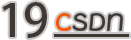k均值（k-means）聚类通常被视为聚类的“入门算法”，其算法原理非常简单。首先从X数据集中选择k个样本作为质心，然后重复以下两个步骤来更新质心，直到质心不再显著移动为止：第一步将每个样本分配到距离最近的质心，第二步根据每个质心所有样本的平均值来创建新的质心。

``````>>> from sklearn import datasets as dss # 导入样本生成器
>>> from sklearn.cluster import KMeans # 从聚类子模块导入聚类模型
>>> import matplotlib.pyplot as plt
>>> plt.rcParams['font.sans-serif'] = ['FangSong']
>>> plt.rcParams['axes.unicode_minus'] = False
>>> X_blob, y_blob = dss.make_blobs(n_samples=[300,400,300], n_features=2)
>>> X_circle, y_circle = dss.make_circles(n_samples=1000, noise=0.05, factor=0.5)
>>> X_moon, y_moon = dss.make_moons(n_samples=1000, noise=0.05)
>>> y_blob_pred = KMeans(init='k-means++', n_clusters=3).fit_predict(X_blob)
>>> y_circle_pred = KMeans(init='k-means++', n_clusters=2).fit_predict(X_circle)
>>> y_moon_pred = KMeans(init='k-means++', n_clusters=2).fit_predict(X_moon)
>>> plt.subplot(131)
<matplotlib.axes._subplots.AxesSubplot object at 0x00000180AFDECB88>
>>> plt.title('团状簇')
Text(0.5, 1.0, '团状簇')
>>> plt.scatter(X_blob[:,0], X_blob[:,1], c=y_blob_pred)
<matplotlib.collections.PathCollection object at 0x00000180C495DF08>
>>> plt.subplot(132)
<matplotlib.axes._subplots.AxesSubplot object at 0x00000180C493FA08>
>>> plt.title('环状簇')
Text(0.5, 1.0, '环状簇')
>>> plt.scatter(X_circle[:,0], X_circle[:,1], c=y_circle_pred)
<matplotlib.collections.PathCollection object at 0x00000180C499B888>
>>> plt.subplot(133)
<matplotlib.axes._subplots.AxesSubplot object at 0x00000180C4981188>
>>> plt.title('新月簇')
Text(0.5, 1.0, '新月簇')
>>> plt.scatter(X_moon[:,0], X_moon[:,1], c=y_moon_pred)
<matplotlib.collections.PathCollection object at 0x00000180C49DD1C8>
>>> plt.show()
``````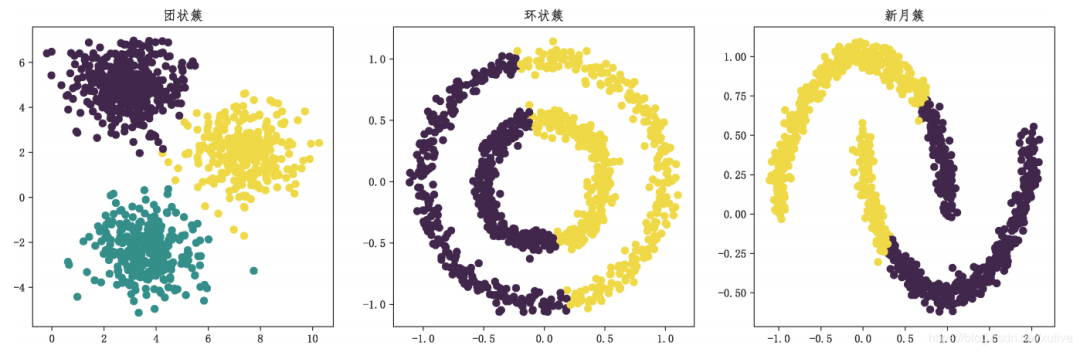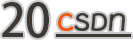DBSCAN类是Scikit-learn聚类子模块cluster提供的基于密度的空间聚类算法，该类有两个重要参数eps和min_samples。要理解DBSCAN 类的参数，需要先理解核心样本。如果一个样本的eps距离范围内存在不少于min_sample个样本（包括这个样本），则该样本称为核心样本。可见，参数eps和min_samples 定义了簇的稠密度。

``````>>> from sklearn import datasets as dss
>>> from sklearn.cluster import DBSCAN
>>> import matplotlib.pyplot as plt
>>> plt.rcParams['font.sans-serif'] = ['FangSong']
>>> plt.rcParams['axes.unicode_minus'] = False
>>> X, y = dss.make_moons(n_samples=1000, noise=0.05)
>>> dbs_1 = DBSCAN() # 默认核心样本半径0.5，核心样本邻居5个
>>> dbs_2 = DBSCAN(eps=0.2) # 核心样本半径0.2，核心样本邻居5个
>>> dbs_3 = DBSCAN(eps=0.1) # 核心样本半径0.1，核心样本邻居5个
>>> dbs_1.fit(X)
DBSCAN(algorithm='auto', eps=0.5, leaf_size=30, metric='euclidean',
metric_params=None, min_samples=5, n_jobs=None, p=None)
>>> dbs_2.fit(X)
DBSCAN(algorithm='auto', eps=0.2, leaf_size=30, metric='euclidean',
metric_params=None, min_samples=5, n_jobs=None, p=None)
>>> dbs_3.fit(X)
DBSCAN(algorithm='auto', eps=0.1, leaf_size=30, metric='euclidean',
metric_params=None, min_samples=5, n_jobs=None, p=None)
>>> plt.subplot(131)
<matplotlib.axes._subplots.AxesSubplot object at 0x00000180C4C5D708>
>>> plt.title('eps=0.5')
Text(0.5, 1.0, 'eps=0.5')
>>> plt.scatter(X[:,0], X[:,1], c=dbs_1.labels_)
<matplotlib.collections.PathCollection object at 0x00000180C4C46348>
>>> plt.subplot(132)
<matplotlib.axes._subplots.AxesSubplot object at 0x00000180C4C462C8>
>>> plt.title('eps=0.2')
Text(0.5, 1.0, 'eps=0.2')
>>> plt.scatter(X[:,0], X[:,1], c=dbs_2.labels_)
<matplotlib.collections.PathCollection object at 0x00000180C49FC8C8>
>>> plt.subplot(133)
<matplotlib.axes._subplots.AxesSubplot object at 0x00000180C49FCC08>
>>> plt.title('eps=0.1')
Text(0.5, 1.0, 'eps=0.1')
>>> plt.scatter(X[:,0], X[:,1], c=dbs_3.labels_)
<matplotlib.collections.PathCollection object at 0x00000180C49FC4C8>
>>> plt.show()
``````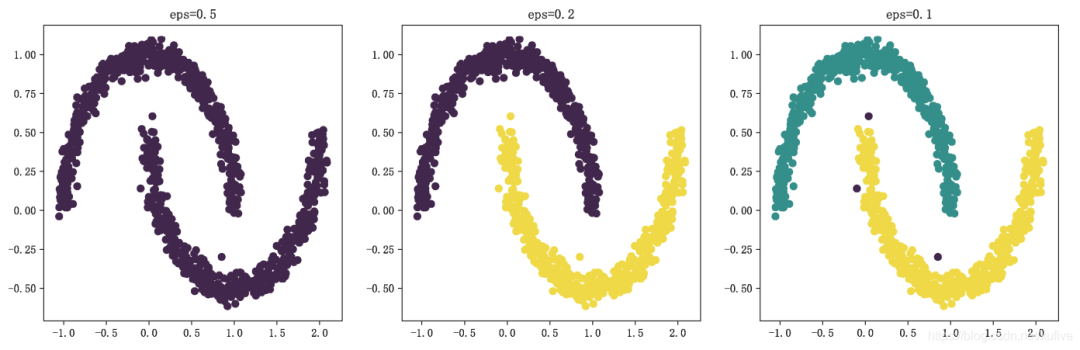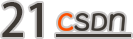``````>>> from sklearn import datasets as dss
>>> from sklearn.decomposition import PCA
>>> ds.data.shape # 150个样本，4个特征维
(150, 4)
>>> m = PCA() # 使用默认参数实例化PCA类，n_components=None
>>> m.fit(ds.data)
PCA(copy=True, iterated_power='auto', n_components=None, random_state=None,
svd_solver='auto', tol=0.0, whiten=False)
>>> m.explained_variance_ # 正交变换后各成分的方差值
array([4.22824171, 0.24267075, 0.0782095 , 0.02383509])
>>> m.explained_variance_ratio_ # 正交变换后各成分的方差值占总方差值的比例
array([0.92461872, 0.05306648, 0.01710261, 0.00521218])
``````

``````>>> m = PCA(n_components=0.97)
>>> m.fit(ds.data)
PCA(copy=True, iterated_power='auto', n_components=0.97, random_state=None,
svd_solver='auto', tol=0.0, whiten=False)
>>> m.explained_variance_
array([4.22824171, 0.24267075])
>>> m.explained_variance_ratio_
array([0.92461872, 0.05306648])
>>> d = m.transform(ds.data)
>>> d.shape
(150, 2)
``````

``````>>> import matplotlib.pyplot as plt
>>> plt.scatter(d[:,0], d[:,1], c=ds.target)
<matplotlib.collections.PathCollection object at 0x0000016FBF243CC8>
>>> plt.show()
``````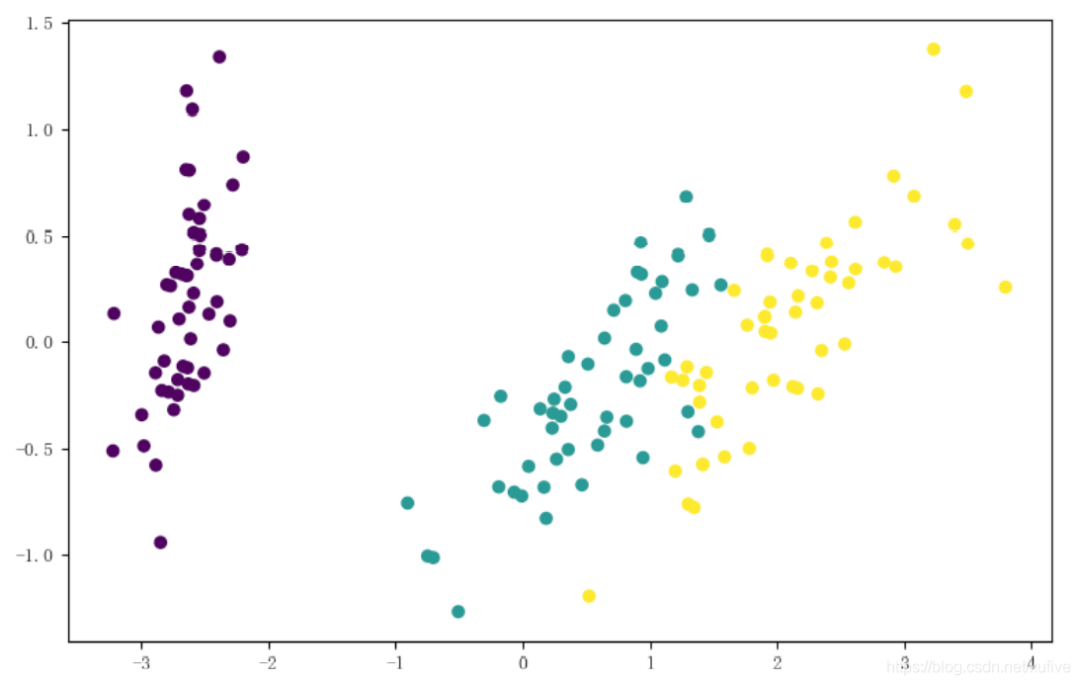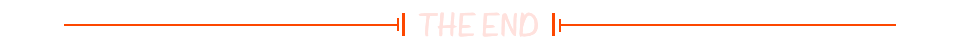``````

``````
``☞新一代 Windows 即将发布、GPT-3 成功商业化，这届微软 Build 大会究竟带来了什么？☞AI时代竟有智能化鸿沟，具备哪些条件才能跨过？☞与 HarmonyOS 拼速度？谷歌正式推出 Fuchsia OS！``20年前，《新程序员》创刊时，我们的心愿是全面关注程序员成长，中国将拥有新一代世界级的程序员。20年后的今天，我们有了新的使命：助力中国IT技术人成长，成就一亿技术人！